# RS Aggarwal Solutions Class 10 Chapter 2 Polynomials MCQS

In this chapter, we provide RS Aggarwal Solutions for Class 10 Chapter 2 Polynomials MCQS Maths for English medium students, Which will very helpful for every student in their exams. Students can download the latest RS Aggarwal Solutions for Class 10 Chapter 2 Polynomials MCQS Maths pdf, free RS Aggarwal Solutions Class 10 Chapter 2 Polynomials MCQS Maths book pdf download. Now you will get step by step solution to each question.

#### RS Aggarwal Class 10 Solutions Chapter 2 Polynomials MCQS

Question 1.
Solution:
(d) √2 x2 – 3√3 x + √6 is polynomial, others are not polynomial.

Question 2.
Solution:
(d) x + 3x is not a polynomial, other are polynomial.

Question 3.
Solution:
(c) Let f(x) = x2 – 2x – 3
= x2 – 3x + x – 3
= x(x – 3) + 1(x – 3)
= (x – 3)(x + 1)
If x – 3 = 0, then x – 3
and if x + 1 = 0, then x = -1
Zeros are 3, -1

Question 4.
Solution:
(b)Question 5.
Solution:
(c)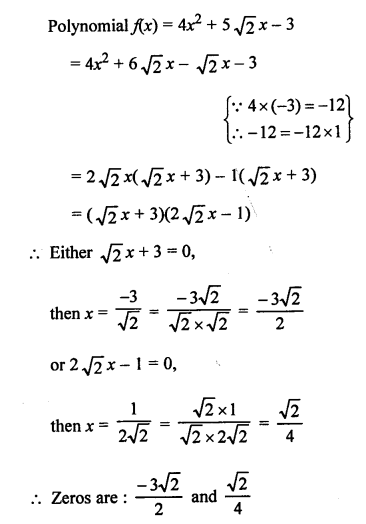Question 6.
Solution:
(b) Polynomial is x2 + 16 x – 2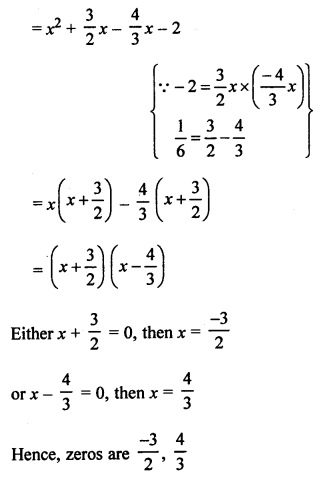Question 7.
Solution:
(a)Question 8.
Solution:
(c) Sum of zeros = 3
Product of zeros = -10
Polynomial : x2 – (Sum of zeros) x + Product of zeros
= x2 – 3x – 10

Question 9.
Solution:
(c) Zeros are 5 and -3
Sum of zeros = 5 – 3 = 2
Product of zeros = 5 x (-3) = -15
Polynomial: x2 – (Sum of zeros) x + Product of zeros
= x2 – 2x – 15

Question 10.
Solution:
(d)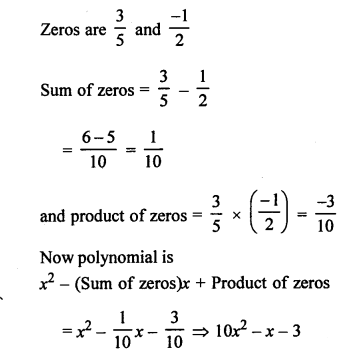Question 11.
Solution:
(b) Let f(x) = x2 + 88x +125
Here, sum of roots = −ba = -88
and product = ca = 125
Product is positive,
Both zeros can be both positive or both negative.
Sum is negative.
Both zeros are negative.

Question 12.
Solution:
(b) α and β are the zeros of x2 + 5x + 8
Then sum of zeros (α + β) = −ba = −51 = -5

Question 13.
Solution:
(c) α and β are the zeros of 2x2 + 5x – 9
Product of zeros (αβ) = ca = −92

Question 14.
Solution:
(d) 2 is a zero of kx2 + 3x + k
It will satisfy the quadratic equation kx2 + 3x + k = 0
k(2)2 + 3x2 + 1 = 0
4k + 6 + k = 0
=> 5k = -6
k = −65

Question 15.
Solution:
(b) -4 is a zero of (k – 1) x2 + 4x + 1
-4 will satisfy the equation (k – 1) x2 + kx + 1 = 0
=> (k – 1)(-4)2 + k(-4) + 1 =0
=> 16k – 16 – 4k + 1 = 0
=> 12k – 15 = 0
=> 12k = 15
=> k = 1512 = 54

Question 16.
Solution:
(c)Question 17.
Solution:
(a)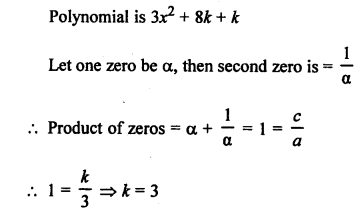Question 18.
Solution:
(d) Polynomial: kx2 + 2x + 3k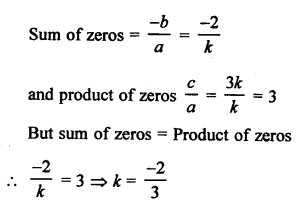Question 19.
Solution:
(b) α, β are the zeros of the polynomial x2 + 6x + 2Question 20.
Solution:
(a) α, β, γ are the zeros of x3 – 6x2 – x + 30
Then αβ + βγ + γα = ca = −11 = -1

Question 21.
Solution:
(a) α, β, γ are the zeros of 2x3 + x2 – 13x + 6, then
αβγ = −da = −62 = -3

Question 22.
Solution:
(c) α, β, γ are the zeros of p(x) such thatQuestion 23.
Solution:
(a)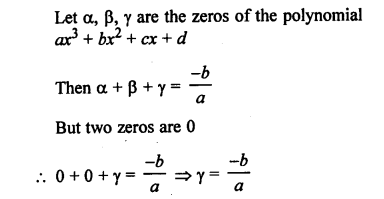Question 24.
Solution:
(b) If one zero of cubic polynomial ax3 + bx2 + cx + d = 0
Let a be zero, thenQuestion 25.
Solution:
(c)Question 26.
Solution:
(d)Question 27.
Solution:
(c) p(x) is divided by q(x), then
p(x) = q(x) x g(x) + r(x)
Either r(x) = 0
Degree of r(x) < deg of g(x)

Question 28.
Solution:
(d) (a) is not a linear polynomial.
(b) is trinomial not binomial.
(c) is not a monomial.
(d) 5x2 is monomial is true.

All Chapter RS Aggarwal Solutions For Class 10 Maths

—————————————————————————–

All Subject NCERT Exemplar Problems Solutions For Class10

All Subject NCERT Solutions For Class 10

*************************************************

I think you got complete solutions for this chapter. If You have any queries regarding this chapter, please comment on the below section our subject teacher will answer you. We tried our best to give complete solutions so you got good marks in your exam.

If these solutions have helped you, you can also share rsaggarwalsolutions.in to your friends.